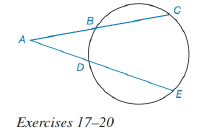Chapter 6.3, Problem 18EElementary Geometry For College St...

7th Edition
Alexander + 2 others
ISBN: 9781337614085

Solutions

Chapter
SectionElementary Geometry For College St...

7th Edition
Alexander + 2 others
ISBN: 9781337614085
Textbook Problem

For Exercises 17 to 2, See Theorem 6.3.6.Given: A C = 12 , A B = 6 , A E = 14 Find: AD.To determine

To find:

The value of AD.

Explanation

Given that, AC=12,AB=6,AE=14.

The diagrammatic representation is given below,

Theorem:

If secant segments are drawn to a circle from an external point, then the products of the length of each secant with the length of its external segment are equal.

By using the theorem 6.3.5 to get the following,

Substitute the values AC=12,AB=6,AE=14 in the above equation to get the following,

Still sussing out bartleby?

Check out a sample textbook solution.

See a sample solution

The Solution to Your Study Problems

Bartleby provides explanations to thousands of textbook problems written by our experts, many with advanced degrees!

Get Started

Find the centroid of the region shown. 13.

Single Variable Calculus: Early Transcendentals

Simplify each of the following. sin8cos8

Trigonometry (MindTap Course List)

For f(x) = ex x, f(x) = a) ex x b) ex 1 c) e1 x d) e1 1

Study Guide for Stewart's Single Variable Calculus: Early Transcendentals, 8th

For , f′(x) =

Study Guide for Stewart's Multivariable Calculus, 8th